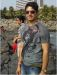﻿ Distance measurement through Microcontroller based distance meter circuit | EngineersGarage

# Distance measurement through Microcontroller based distance meter circuit

4 posts / 0 new
Sumanta Kumar ShowOffline
Last seen: 7 years 4 days ago
Joined: 04/05/2012 - 11:43
Distance measurement through Microcontroller based distance meter circuit

Myself Sumanta Kumar Show is trying to implement a program regarding measurement of Distance through AT89C2051 Microcontroller. The program is as follows -

;Program listing:

;\$mod51
ORG 0H
AJMP 30H
ORG 0BH ;TIMER 0 INTERRUPT VECTOR; AJMP TIMER0ISR ;Timer 0 Interrupt service routine address

ORG 30H
MOV SP,#60H ;set stack pointer
MOV P3,#0FFH ;set all port 3 bits high to enable inputs also
MOV P1,#03 ;set port 1 to all zeros expect bits 0,1

MOV TMOD,#01100001B ;TIMER 1 - MODE 2 COUNTER,TIMR-0 TO MODE 1
BEG: MOV TH0,#0H ;TIMER REG.0 IS SET TO 0, GIVES 64ms
MOV TL0,#0 ; timer low reg. is also so
;TOTAL CYCLE TIME IS 64.6ms ,350m/s gives 0.35mx65=22.5m
; up and down 10 metres say! .35 m/ms,.35 mm/us, 1mm per 3 micros
; up and down .35/2 mm/us = 1/6 mm/us
; VELOCITY OF SOUND IN AIR IS 350 M/S
; AFTER 100 TIMES, WE HAVE TO STOP TRANSMITTING FOR A TIME OF ABOUT .1 S
; SO WE STOP FOR THIS AMOUNT OF TIME and expect an echo.
mov r2,#25 ; 25 pulses 26 us =.53 ms(343m/s*.5ms=17cm)

pulse: setb p3.4 ;generates 40KHz
mov r1,#5
djnz r1,\$
clr p3.4
mov r1,#5
djnz r1,\$ ;wait for 13 us
djnz r2, pulse ;20pulses
setb tr0 ;start timer

mov r2,#10
djnz r2,\$ ;wait 20 us
check_echo:
jnb p3.6,checktimeout
MOV 40h,TL0 ; read timer count
MOV 41h,TH0
mov r0,40h
mov r1,41h
mov r3,#0
mov r2,#6
call UDIV16 ;divide by 6
mov 40h,r0
mov 41h,r1
mov 50h,#25
disp: call disp1 ; show the value on LED

djnz 50h,disp ; so many times for a visible time limit

jmp beg
checktimeout: mov a,th0
cjne a,#0c0h,check_echo ;upto 4 metres

jmp beg
;subroutine UDIV16
;16 bit/16bit unsigned divide
;input r1,r0 =dividend X
;input r3,r2 =divisor Y
;output r1,r0 =quottient q of x/y
;output r3,r2 = remainder
; alters acc,r4-47,flags,dptr
UDIV16: mov r7,#0 ;clear partial remainder
mov r6,#0 ;
mov B,#16 ;set loop count
div_loop: clr C ;clear carry flag
mov a,r0 ; shift the highest bit of dividend into

rlc a
mov r0,a
mov a,r1
rlc a
mov r1,a
mov a,r6 ;... the lowest bit of partial remainder

rlc a
mov r6,a
mov a,r7
rlc a
mov r7,a
mov a,r6
clr C
subb a,r2
mov dpl,a
mov a,r7
subb a,r3
mov dph,a
cpl C
jnc div_1 ;update partial reaminder if borrow

mov r7,dph
mov r6,dpl ; update parital reminder
div_1: mov a,r4
rlc a
mov r4,a
mov a,r5
rlc a
mov r5,a
djnz B,div_loop
mov a,r5
mov r1,a ; put qt. in r0,r1
mov a,r4

mov r0,a
mov a,r7 ;get rem. saved before the
mov r3,a ;last subtraction.
mov a,r6
mov r2,a
ret
;16 Bit Hex to BCD Conversion for 8051 Microcontroller

; This routine is for 16 bit Hex to BCD conversion
;
;Accepts a 16 bit binary number in R1,R2 and returns 5 digit BCD in

;R7,R6,R5,R4,R3(upto 64K )
Hex2BCD: ;r1=high byte ;r7 most significant digit

;R2 = LSByte
MOV R3,#00D
MOV R4,#00D
MOV R5,#00D
MOV R6,#00D
MOV R7,#00D
MOV B,#10D
MOV A,R2
DIV AB
MOV R3,B ;
MOV B,#10 ; R7,R6,R5,R4,R3
DIV AB
MOV R4,B
MOV R5,A
CJNE R1,#0H,HIGH_BYTE ; CHECK FOR HIGH BYTE

SJMP ENDD
HIGH_BYTE: MOV A,#6
MOV B,#10
DIV AB
MOV R3,B
MOV B,#10
DIV AB
MOV R4,B
MOV B,#10
DIV AB
MOV R5,B
SJMP CONTINUE
CONTINUE: MOV R6,A
DJNZ R1,HIGH_BYTE
MOV B, #10D
MOV A,R6
DIV AB
MOV R6,B
MOV R7,A
ENDD: ret
DISP1:
REFRESH: ; content of 18 to 1B memory locations are output on LEDs

; only numbers 0 to 9 and A to F are valid data in these locations

mov r1,41h
mov r2,40h
CALL HEX2BCD
MOV 18H,r3 ; least significant digit
MOV 19H,r4 ; next significant digit
MOV 1AH,r5

MOV 1BH,R6 ; most significant digit(max:9999)

refresh1: MOV R0,#1bh ; 1b,1a,19,18, holds values for 4 digits

MOV R4,#8 ; pin p3.3_ 0 made low one by one starts wth 18

mov r7,#2 ; decimal pt.on 3rd digit from left (2 nd fromright)

PQ2: CALL SEGDISP
deC R0
mov a,r4
rrc a
mov r4,a
jnc pQ2
PV3:
RET
SEGDISP:
mov dptr,#ledcode
MOV A,@R0
ANL A,#0FH
MOVC A,@A+dptr
segcode:
MOV R5,A
ORL A,#03H ; WE WANT TO USE PORT 1 BITS 0 AND 1 FOR INPUT ANLOG

; so retain them high
S3: MOV P1,A ; SEGMENT_PORT
MOV A,R5 ;we use p3.7 for the segment ‘a’ of display

RRC A ;so get that bit D0into carry
; cpl c
; mov p3.5,c ; dec pt is D0 bit that is wired to p3.5

rrc a
mov p3.7,c ;segment ‘a;
S1: MOV A,R4 ; get digit code from r4 00001000

cpl a ;11110111
rrc a ;11111011-1
mov p3.0,c ; output to drive transsitors for digit lighting

rrc a ;11111101-1
mov p3.1,c
rrc a ;11111110-1
mov p3.2,c
rrc a ;1111111-0 yes low makes leftmost digit show msdigit

mov p3.3,c
S5:
S4: ACALL DELAY1 ; let it burn for some time

MOV A,#0ffH ; extinguish the digit after that time

MOV P3,A ; to prevent shadow
s6: RET
ledcode:
DB 7EH,0CH,0B6H,9EH,0CCH,0DAH,0FAH
DB 0EH,0FEH,0CEH,0EEH,0F8H,72H,0BCH,0F6H,0E2H

;these are code for the numbers 0 to 9 and A to F

DELAY1: MOV R1,#0ffH
N: NOP
DJNZ R1,N
RET
END

The corresponding circuit is given in the Attachment. I have burned the program into the microcontroller, but still the circuit is not working and no display is coming in 7 segment display (LTS542). Please check the program and the circuit and suggest me how to rectify the problem. Yours help will be highly appreciated.

Sumanta

Anonymoushi this is not to big deal

AmrithOffline
Last seen: 6 years 4 months ago
Joined: 23/04/2012 - 16:57

Hi Sumanta,